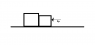# Reaction Force: A Comparison of Masses

• Redfire66

## Homework Statement

Two blocks with different masses are in contact on a frictionless surface. A force, Fp can be applied to either block. If the magnitude of Fp is the same on both cases, the force exerted by the heavier block on the lighter box is
A) The same
B) Greater when force Fp is applied on the lighter block
C) Greater when force Fp is applied on the larger block

F = ma

## The Attempt at a Solution

So I'm assuming that they mean the reaction force, also would the acceleration be the different for each mass? Since the total force would equal Fp and so Fp = ma, which means that the smaller mass would have a larger acceleration but that doesn't explain to me how the reaction force is different. I also drew a diagram but that didn't really help me.
When I looked at it, I first assumed they would be the same since I thought the reaction force would both equal Fp no matter what. I have the answer, however I'm not really sure I understand the concept behind it (the answer is B)
I made the smaller box look smaller just so I can visualize it easier in the diagram provided.

#### Attachments

•Reaction Force.png
275 bytes · Views: 416
Resist the temptation to call the force between the boxes a reaction force. It is a normal force whose magnitude is determined from Newtons laws. Since the blocks remain in contact, their accelerations must be the same. Draw free body diagrams of both blocks and each block separately, solve for the unknown forces, and compare for each case.

|Never mind figured it out

Last edited: